Functions are used throughout mathematics and have applications in many disciplines.  However, we are often interested in finding the extreme values, such as function maximums.

So, what do you need to know about function maximums?  A function can have multiple local maximum values, but it can have only one absolute (or global) maximum value.  However, the maximum value (a y-value) can occur at more than one x-value.  Every absolute maximum is a local maximum, but not every local maximum is an absolute maximum.

Of course, certain functions may only have one local maximum, and others may have no absolute maximum.

Let’s get started.

## 7 Common Questions About Function Maximums

A function can have multiple local maximum values, but it can have only one absolute (global) maximum value.  However, the maximum value (a y-value) can occur at more than one x-value.

This still raises the question of when a local maximum is an absolute maximum, so we’ll start there.

### Can A Local Maximum Be An Absolute Maximum?

A local maximum can be an absolute maximum, and every absolute maximum is also a local maximum. However, not every local maximum is an absolute maximum.

The Venn diagram below illustrates this idea: there are always at least as many absolute maximums as there are local maximums for a function.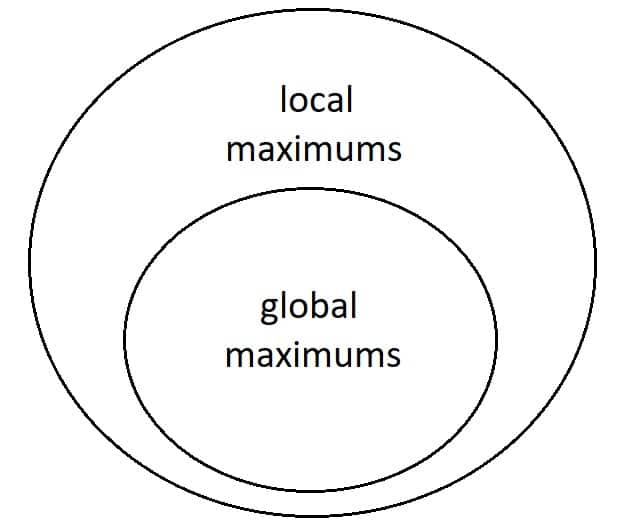This Venn diagram shows the relationship between local and global (absolute) maximums. Every global maximum is a local maximum, but not every local maximum is a global maximum.

Let’s look at an example to make this idea more clear.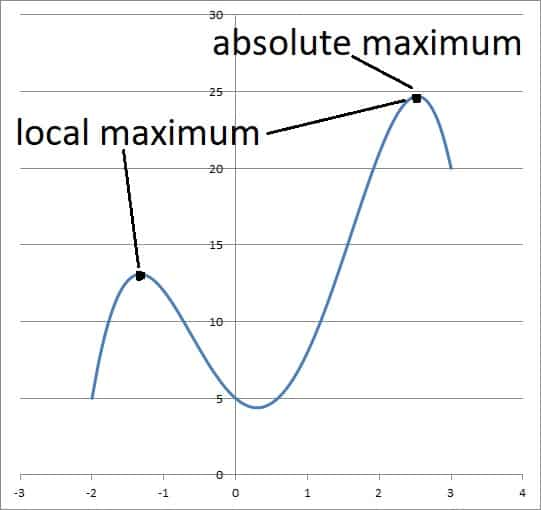This graph shows a function and its two local maximums (the one on the right is an absolute or global maximum).

As you can see, there are two local maximums on this graph.  However, the one on the right has the higher y-value, so it is also an absolute maximum for the function.

### Does A Linear Function Have A Maximum?

A linear function does have a maximum in some cases (when we restrict its domain).  However, a linear function may not have a maximum if the domain is unbounded.

For example, the function f(x) = x is unbounded on the set of real numbers.  The reason is that we can always plug in a larger value of x to get a larger output (y-value).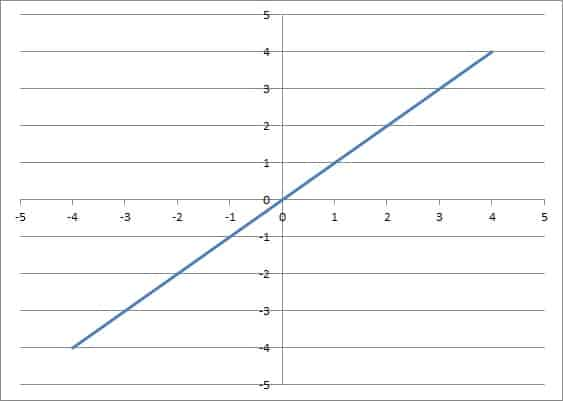The linear function f(x) = x has no maximum value on the set of real numbers.

However, this same function has a maximum of y = 5 at x = 5 on the domain [0, 5].

Remember that for a linear function with a maximum, the maximum will occur:

• At the right endpoint if the slope is positive.
• At the left endpoint if the slope is negative.
• At all points if the slope is zero.

For example, the linear function g(x) = -5x on the domain [-4, 4] has a maximum value of 20 at its left endpoint, x = -4.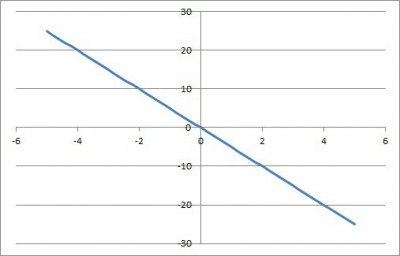The line f(x) = -5x has a maximum value of y = 20 at the left endpoint x = -4 on the domain [-4, 4].

### Does Every Function Have An Absolute Maximum?

Not every function has an absolute maximum.  As mentioned above, some linear functions do not have a maximum value if the domain is unrestricted.

There are other functions with no absolute maximum if their domains are unrestricted.

For example, the quadratic function y = x2 + 1 (whose graph is a parabola, pictured below) has no absolute maximum on the set of real numbers.  The reason is that we can always plug in larger x values to get larger outputs (y-values).

There are even functions that have no absolute maximum on a bounded domain.  For example, the function f(x) = 1/x on the domain [0, 1] has no global maximum.

The reason is that 1/x is unbounded as x approaches zero.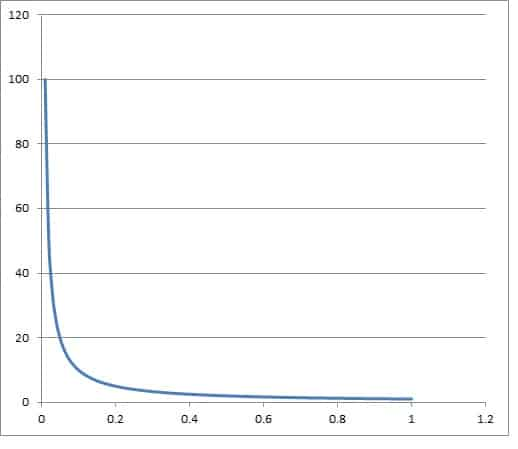The function f(x) = 1/x has no maximum on the interval [0, 1] because the value of f(x) becomes ever larger as x approaches zero.

### Can A Maximum Be Negative?

A maximum can be negative in some cases.  For example, the quadratic function f(x) = -x2 – 3 has an absolute maximum value of y = -3 at x = 0 (the vertex of the parabola).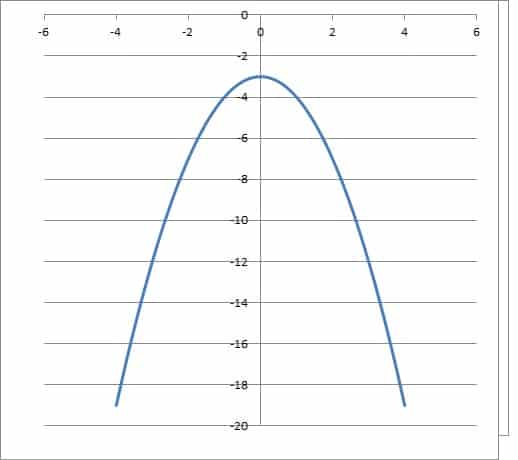the quadratic function f(x) = -x2 – 3 has an absolute maximum value of y = -3 at x = 0 (the vertex of the parabola).

This maximum (a y-value) is negative.  We can also have maximums where the x values are negative.

For example, the function g(x) = -(x + 1)2 – 4 has an absolute maximum value of y = -4 at x = -1 (the vertex of the parabola).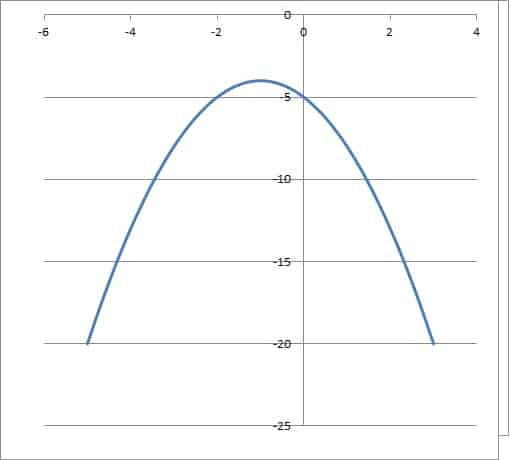The quadratic function g(x) = -(x + 1)2 – 4 has an absolute maximum value of y = -4 at x = -1 (the vertex of the parabola).

### Can A Maximum Be An Inflection Point?

A maximum can also be an inflection point.  We can come up with a piecewise function with a point that satisfies both of these conditions.

For example, consider the piecewise function: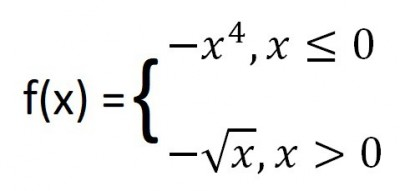This piecewise function is continuous and differentiable at x = 0 and has both a maximum and an inflection point there.

This piecewise function is pictured below.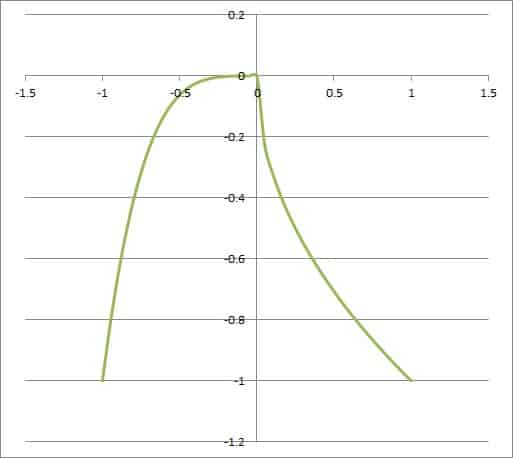This piecewise function is continuous and differentiable at x = 0 and has both a maximum and an inflection point there.

Note that:

• This function is continuous at x = 0 (both functions have a limit of y = 0 as x approaches 0, and the value of y is 0 at x = 0). (You can learn more about zero limits in my article here).
• The first derivative is zero at x = 0, and it changes signs from positive to negative at x = 0, so we have a function maximum there.
• Finally, the second derivative is zero at x = 0, and it changes signs from negative to positive at x = 0, so we have an inflection point there as well.

### Can A Quadratic Function Have A Maximum & Minimum?

A quadratic function on the set of real numbers has either a maximum or a minimum, but not both.  However, a quadratic function can have a maximum and a minimum on a restricted domain.

For example, consider the quadratic function

• g(x) = x2

defined on the interval [1, 2].

The minimum value of the quadratic function on this interval is y = 1, when x = 1.  The maximum value of the quadratic function on this interval is y = 4, when x = 2.

The graph below helps to illustrate this.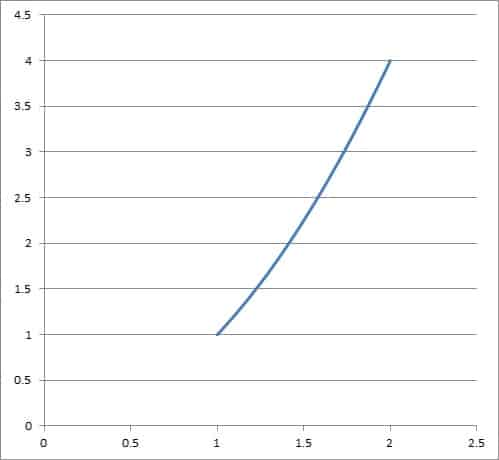The quadratic function g(x) = x2 has a maximum value of 4 at x = 2 (right endpoint) and a minimum value of 1 at x = 1 (right endpoint) when restricted to the interval [1,2].

This still leaves the question of whether a quadratic function has a maximum or a minimum when defined on the entire set of real numbers.

#### How To Tell If A Quadratic Function Has A Maximum Or Minimum

Remember that a quadratic function has the form

• f(x) = ax2 + bx + c

where a is not zero.

The value of a determines the nature of the quadratic function’s maximum or minimum over the real numbers:

• If a is positive, then the parabola is concave up (opens up), and the quadratic function has a minimum at the vertex of the parabola.  However, it has no maximum value.
• If a is negative, then the parabola is concave down (opens down), and the quadratic function has a maximum at the vertex of the parabola.   However, it has no minimum value.

For example, the graph of the parabola f(x) = x2 – 1 (shown below) has a minimum of y = -1 at x = 0. It has a minimum since the leading coefficient has a value of 1, which is positive (a > 0).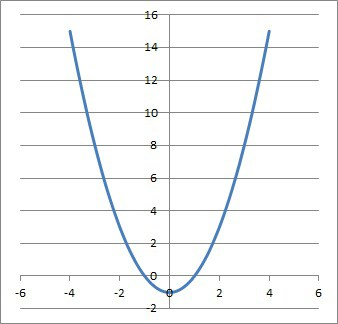The graph of the parabola f(x) = x2 – 1 has a minimum of y = -1 at x = 0. It has a minimum since the leading coefficient has a value of 1, which is positive (a > 0).

On the other hand, the graph of the parabola f(x) = -x2 – 1 (shown below) has a maximum of y = -1 at x = 0. It has a maximum since the leading coefficient has a value of -1, which is negative (a < 0).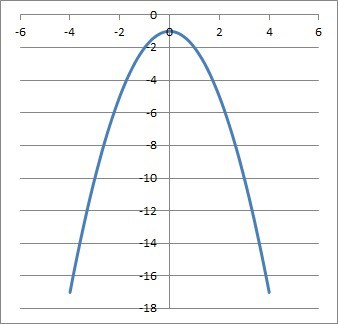The graph of the parabola f(x) = -x2 – 1 has a maximum of y = -1 at x = 0. It has a maximum since the leading coefficient has a value of -1, which is negative(a < 0).

The table below summarizes the relationship between the sign of a in a quadratic function and the nature of the extreme values (maximum or minimum) of a parabola.

ax2 + bx + c

## Conclusion

Now you know more about function maximums, along with when and how they can occur.  You also know the answers to some common questions about function maximums.

You can learn about how to use derivatives and graphs to find function maximums here.